# Lab presentation .pdf

### File information

This PDF 1.5 document has been generated by MicrosoftÂ® Office PowerPointÂ® 2007, and has been sent on pdf-archive.com on 09/02/2012 at 21:17, from IP address 79.55.x.x. The current document download page has been viewed 1613 times.
File size: 1.66 MB (33 pages).
Privacy: public file

### Document preview

Modeling, Verification and Control
of Asynchronous Motor
(Induction Motor)
Vahab Rostampour
Nihat Ozlü
Department of Automation and Control
Engineering, Politecnico di Milano, Italy

Laboratory supervisor:
Dr. Alan Facchinetti
Dr. Alberto Bezzolato

Outline
 Introduction
 Induction Motor Model
 Estimators
 Controllers
 Verification
 Controller *
 Observers *
 Conclusion

2

* Fulfill in theory base

Introduction

3

• Due to enhanced and improved design of microprocessors and
DSPs and their computational efficiency in the field of Electrical
drive control also their compatibility with each other enable to
achieve great success in the efficient control of AC drive with
improved results in power dissipation.
• Thanks to the very well known vector controls schemes which
led to these achievements (Field Oriented Control-FOC).
• In spite of linear dynamical systems, observability analysis is a
very difficult task for nonlinear dynamical systems.
• Observable systems, linear or nonlinear, do not show same
behaviors with different observers.
• Estimating the state of some systems are easier than other
systems with a same type of observer.

Model of Induction motor

4

• The objective of Field Orientated Control (FOC) is to
control the stator currents represented by a vector.
The control is based on the creation of a time invariant
system which works on two coordinates namely (d and
q coordinates). Transformation is required to convert a
three phase time dependent system into a system of
d-q axis.
• This transformation results a control in which the two
components (d-q) of stator current acts separately on
the rotor flux and torque and thus the FOC control
allows us to control the induction motor.

5

Theoretical View

Model of Estimatores
• Current-Voltage Estimator

Theoretical
View

The inputs used to design this estimator are stator voltages and currents only. Flux of
stator is calculated from integration operation on voltages as shown below, and the rotor
flux is calculated from stator currents and stator flux calculated.

6

View

Model of Estimatores
• Current-Speed Estimator

Theoretical
View

The inputs for this type of estimator are phase current and mechanical speed. The
design of inverter is shown below

7

View

Model of Estimatores
• Double Estimator

View

The two observers can be joined through a closed-loop leading to a new observer that
minimizes the errors of the two original observers.

8

Model of Controllers
• Current Loop Controller

Theoretical
View

The closed loop transfer function:

9

View

Model of Controllers
• Speed Loop Controller

Theoretical
View

The closed loop transfer function:

10

View

Verification
• Model results:

Torque

11

Speed

Verification
• Estimators results:

12

Verification
• Controllers results:

Vq,d

13

Vq,d (es)

Classical Controller (PID)
• Current Controller (inner loop):
num = [0 1];
den = [Lks Rks];
G1 = tf(num,den); %plant, open loop system
Fc=100; %[Hz]
Wc=2*pi*Fc;
Kp_i=Wc*Rks;
Ki_i=(Rks*Kp_i)/Lks;
T_i=Kp_i/Ki_i;
num=[Kp_i*(T_i) Kp_i];
den=[1 0];
C=tf(num,den); % Controller
CL = feedback(G1*C,1); %closed loop system

14

15

Classical Controller (PID)
• Speed Controller (outer loop):
num = [0 1];
den = [J 0];
G2 = tf(num,den); %plant
Fw=10; %[Hz]
Ww=2*pi*Fw;
Kp_w=Ww;
Ki_w=(Kp_w)/J;
T_w=Kp_w/Ki_w;
num=[Kp_w*(T_i) Kp_w];
den=[1 0];
C2=tf(num,den); % Controller
CL2 = feedback(G2*C2,1); %closed loop system

16

17

Model Base Predictive Control

18

Continuous time LTI systems
 x(t )  Ax(t )  Bu (t )

 y (t )  Cx(t )  Du (t )

System’s model:
Luenberger observer:

xˆ  Axˆ  L( y  Du  Cxˆ )  Bu

Theorem 1. In the design of Luenberger observer if
the observer poles are considered in different places:
a) Estimation error energy caused by measurement
errors has an upper bound which depends on the
norm of the observer gain:
xˆ

19

2

 y

 (P )
2

Min Re al (aˆi )
i

L

2

Continuous time LTI systems
b) Estimation error energy caused by model uncertainties
in matrix A has an upper bound presented below:
e (t ) 2  A

 P 
2

  P1  B

2

Min Re al (aˆi ) Min Re al (ai )
i

i

A  P11D1P1 , D1  diag (ai )
Aˆ  A  LC  P 1DP , D  diag (aˆi )

  P1  : condition number of P1
  P  : condition number of P

20

u (t )

2

Discrete time LTI systems
 xk 1  Axk  Buk

 yk  Cxk  Duk

System’s model:
Luenberger observer:

xˆk 1  ( A  LC ) xˆk  L( yk  Duk )  Buk

Theorem 2. In the design of Luenberger observer if
the observer poles are considered in distinct places:
a) Estimation error energy caused by measurement
errors has an upper bound which depends on the
norm of the observer gain:
xˆ k

21

2

L

2

Min 1  aˆi
i

y k

2

Discrete time LTI systems
b) Estimation error energy caused by model uncertainties
in matrix A has an upper bound presented below:
e k

2

 A

 P 

2

Min 1  aˆi
i

  P1  B

2

  Min 1  a     P  A
i

i

1

A  P11D1P1 , D1  diag (ai )
Aˆ  A  LC  P 1DP , D  diag (aˆi )

  P1  : condition number of P1
  P  : condition number of P

22

2

uk

2

Kalman filter: an optimal estimator
 Dependence of the observer gain on the observer poles
 Dependence of the upper bound on the observer poles
 An optimization criteria is required to specify the observer poles
Kalman filter is an optimal estimator:
Process model:
Measurement model:
Assumptions:
Initial conditions:
Covariance updating:
Kalman gain:
Estimation updating:

23

x(t )  Ax(t )  G (t )
z (t )  Hx(t )  v(t )
x(0) ( x0 , P0 ),  (0, Q), v
P(0)  P0 , xˆ (0)  x0

(0, R)

P(t )  AP  PAT  PH T R1HP  GQGT
K (t )  P(t ) H T R 1
xˆ (t )  Axˆ (t )  K (t )  z(t )  Hxˆ(t )

Kalman filter for discrete systems
Process model:
Measurement model:

Assumptions:
Initial conditions:
Time updating:

xk 1  Ak xk  Bk uk  Gk k
zk  H k xk  vk
x0

( x0 , Px0 ), k

(0, Qk ), vk

(0, Rk )

P0  Px0 , xˆ0  x0

xˆk1  Ak xˆk  Bk uk

Pk1  Ak Pk AkT  Gk Qk GkT
Measurement updating:

24

 

Pk 1   P


k 1

1

 H R H k 1 

T
1
k 1 k 1

1

xˆk 1  xˆk1  Pk 1H kT1Rk11 zk 1  H k 1 xˆk1

Comparative observability index
Definition 1. Consider continuous time LTI systems with
model uncertainties and measurement errors which are
modeled in the form:
x(t )  ( A  A) x(t )  Bu (t )  G (t )

y(t )  Cx(t )  y(t )  v(t )
Suppose that the steady state Kalman filter is designed to
estimate the state of these systems. Comparative
observability Index of these systems are defined as:

  P1 
 (P )
  L   B A
1
2
2
2
2
Min Re al (aˆi ) 
Min Re(ai )    P1  A 2
i
i

A system is more observable than other systems if it has
less observability Index.

25

Comparative observability index
Definition 2. Consider discrete time LTI systems with model
uncertainties and measurement errors which are modeled
in the form:
xk 1  ( A  A) xk  Buk  Gk

yk  Cxk  yk  vk
Suppose that the steady state Kalman filter is designed to
estimate the state of these systems. Comparative
observability Index of these systems are defined as:

 (P )
 L   B
1
2
2
Min  1  (aˆi )  
i

26

 (P1 )

2

 Min 1  a     P  A 
i

i

1

2

A 2 

A system is more observable than other systems if it has
less observability Index.

Generalization to nonlinear
systems based on EKF
Process model:
Measurement model:
Assumptions:
Initial conditions:

x  f  x, u, t   G (t )
z  h  x, t   v
x(0)

 x0 , P0  ,  (t )  0, Q  , v(t )  0, R 

P(0)  P0 , xˆ (0)  x0

Covariance updating:

P  A  xˆ, t  P  PAT  xˆ, t   GQGT  PH T  xˆ, t  R 1H  xˆ, t  P
A  x, t  

Kalman gain:
Estimation updating:

27

f  x, u, t 

x
K  PH T  xˆ, t  R 1

, H  x, t  

h  x, t 
x

Implementation??

xˆ  f  xˆ, u, t   K  z  h  xˆ, t 

Generalization to nonlinear
systems based on EKF
The structure of
the EKF is:

28

 P  A  xˆ, t  P  PAT  xˆ, t   GQG T  PH T  xˆ, t  R 1H  xˆ, t  P

T
1
ˆ
ˆ
ˆ
 z  z  h  xˆ, t  
x

f
x
,
u
,
t

PH
x
,
t
R


P(0)  P0 , xˆ (0)  x0
y  xˆ

 Steady state filter is meaningless.
 We are not easily able to find an
upper bound for the estimation error due
to measurement error.
 Comparing the observability of
nonlinear systems with EKF based on
less sensitivity to measurement error is
definable but is not easily computable.

Changing the
structure of
the observer

H-infinity filtering
Different views on H-infinity filtering:
1) H-infinity optimal controller
2) Minimizing the energy of exogenous signals in the estimation
of variables
The first view:

J : sup

L2 0, 

29

z  zˆ

2
2

2
2

2

H-infinity filter: a robust estimator
Second view:
Cost function:

Suboptimal condition:

Estimation updating:
Filter gain:

J E

 

T
e
 edt  E

x(0)  xˆ (0)

2
P01

  x  xˆ   x  xˆ  dt
T

x  xˆ S dt

2

 

2
Q 1

dt   v

2
R 1

2
dt

xˆ  Axˆ  Bu u  K (t )  y  Cxˆ 
K (t )  P(t )C T D T R 1D 1

Covariance updating:

P(t )  AP(t )  P(t ) AT  B QBT  K (t )CP(t )

30

P(t )  AP(t )  P(t ) AT  B QBT  K (t )CP(t )   2 P(t ) SP(t )

Conclusion

31

32
Torque
Speed

33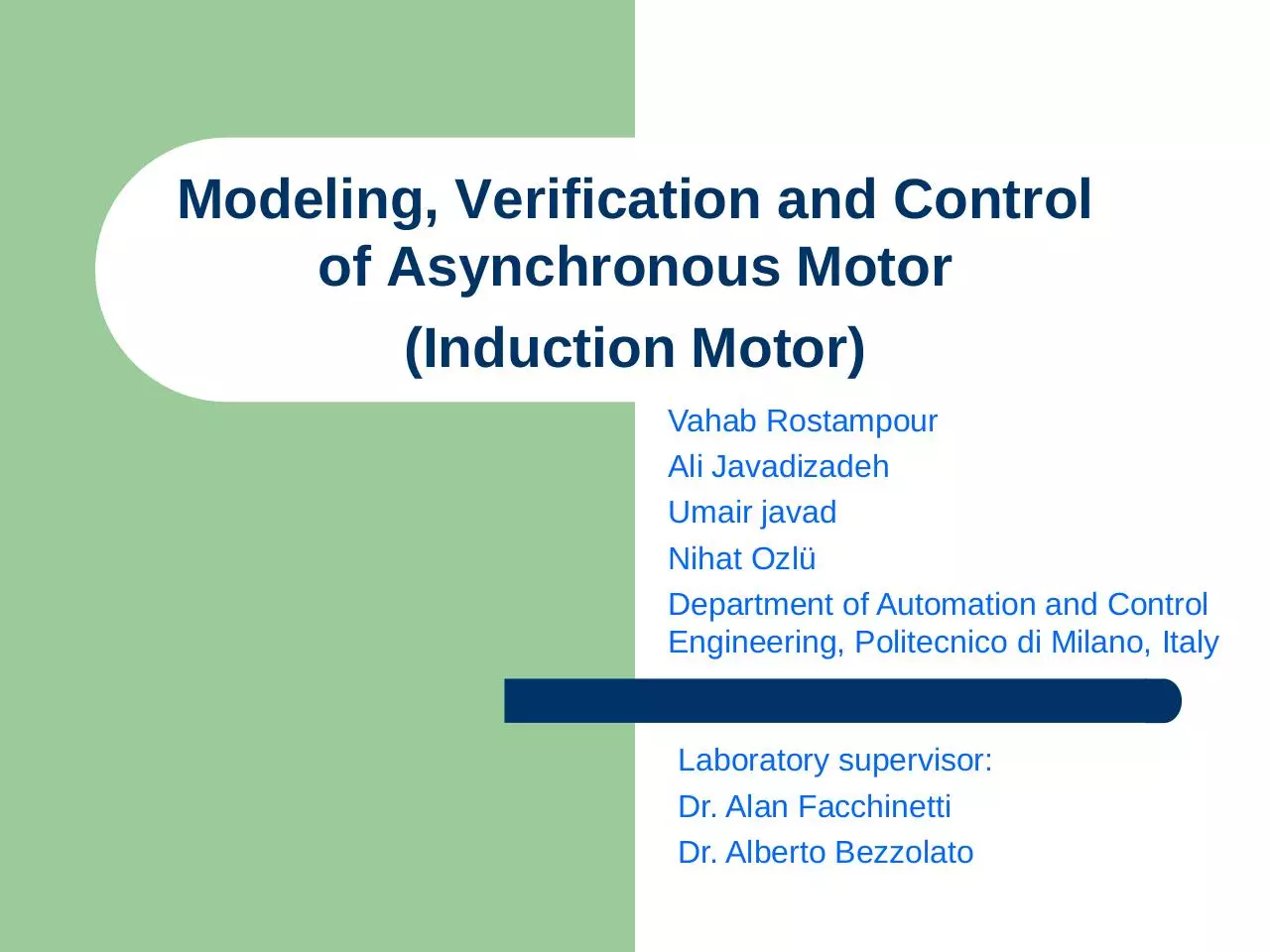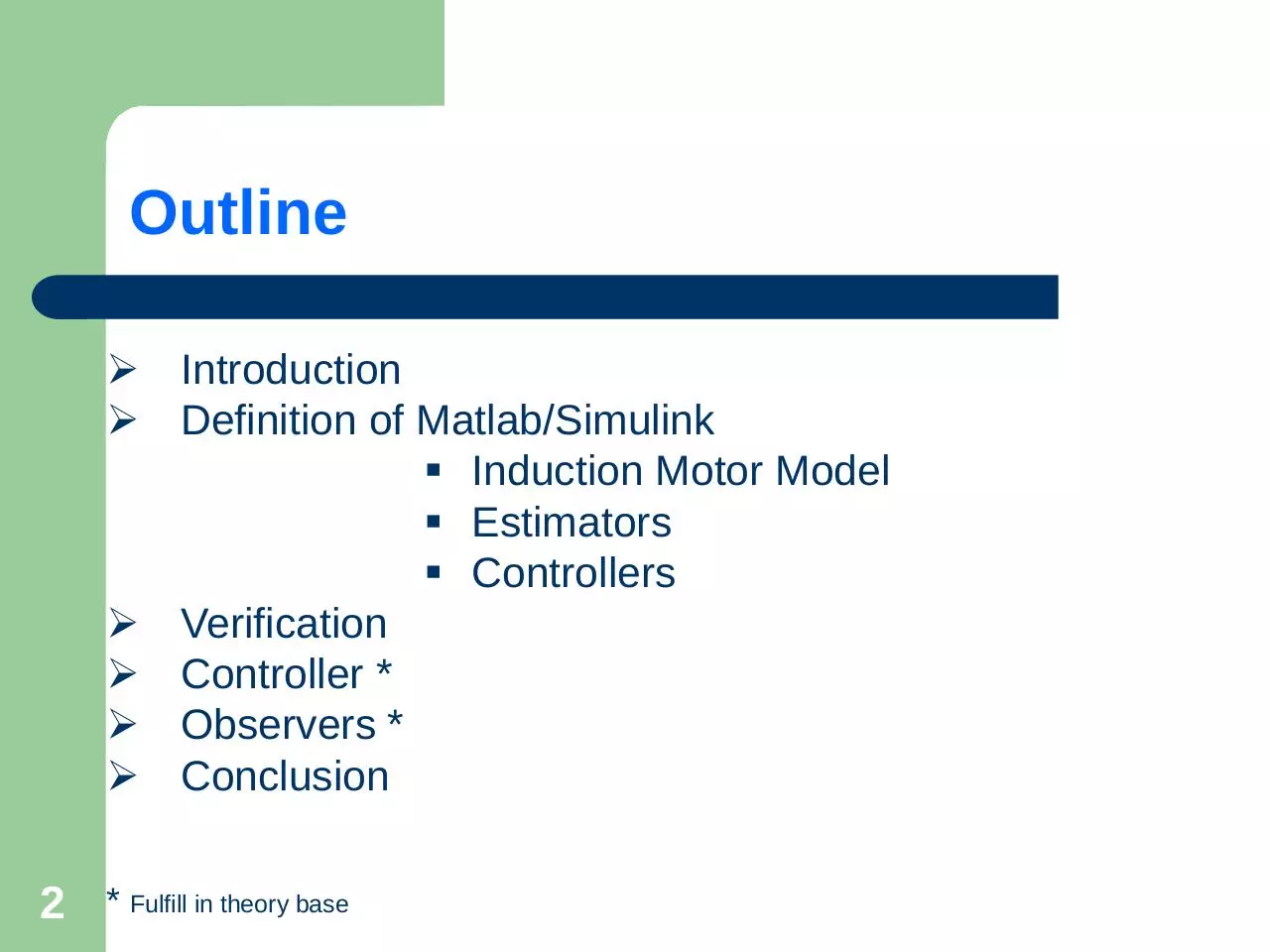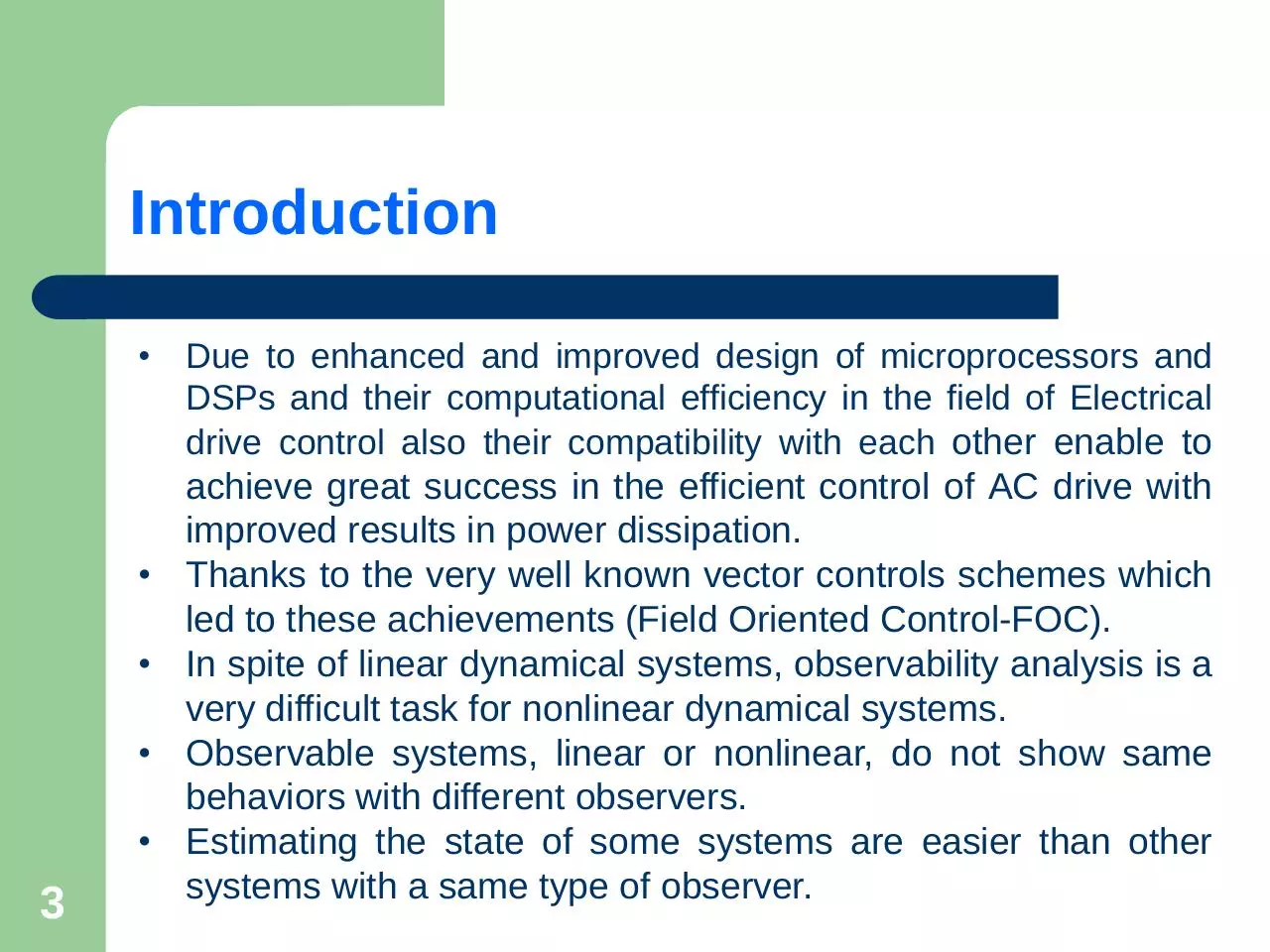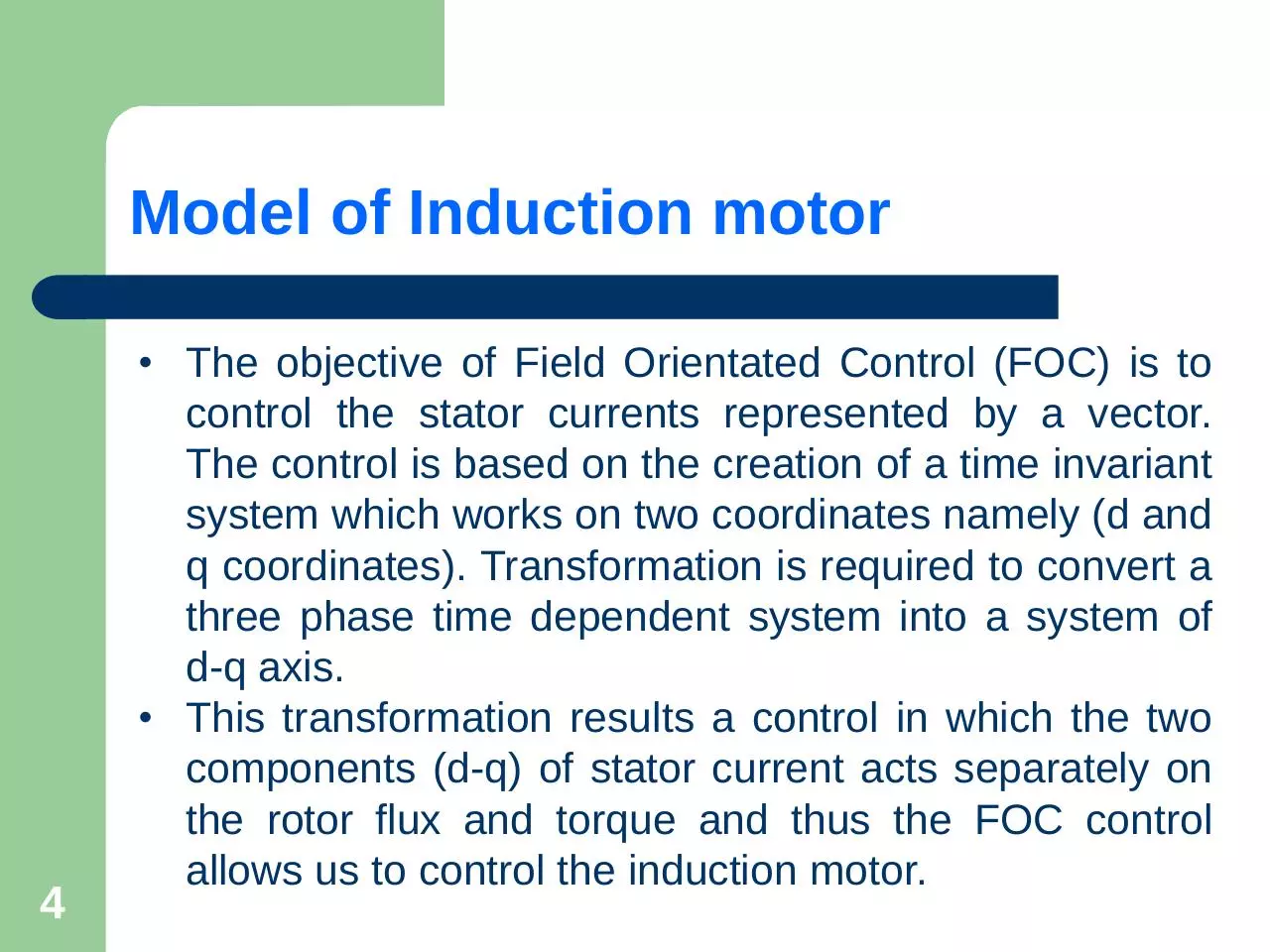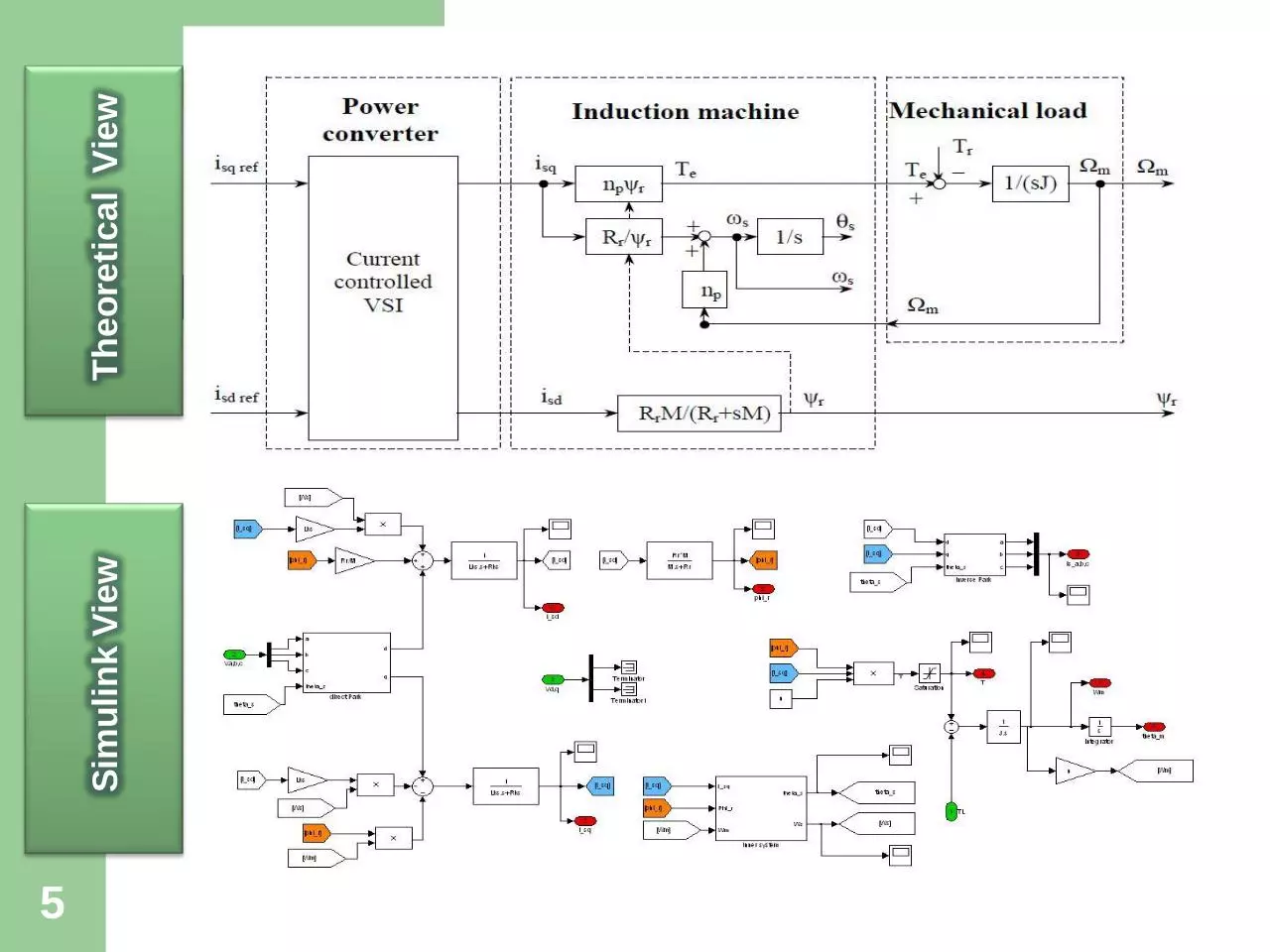Lab_presentation.pdf (PDF, 1.66 MB)

### Share on social networks

#### HTML Code

Copy the following HTML code to share your document on a Website or Blog